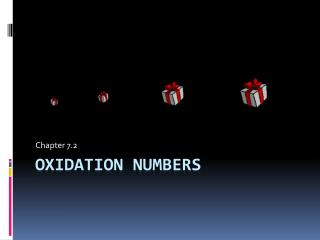DownloadDownload PresentationOxidation numbers

# Oxidation numbers

Télécharger la présentation## Oxidation numbers

- - - - - - - - - - - - - - - - - - - - - - - - - - - E N D - - - - - - - - - - - - - - - - - - - - - - - - - - -
##### Presentation Transcript

1. Chapter 7.2 Oxidation numbers

2. Objectives: • List the rules for assigning oxidation numbers • Give the oxidation number for each element in the formula of a chemical compound. • Name binary molecular compounds using oxidation numbers and the Stock system.

3. Oxidation Numbers or oxidation state • Assigned in order to indicate the general distribution of electrons among the bonded atoms in a molecular compound or polyatomic ion • Only needed for molecules or polyatomic ions • Ionic compound have charges

4. Assigning Oxidation Numbers • General rule • Shared electrons are assumed to belong to the more electronegative atom • Atoms in a pure element • Oxidation number equals zero Ex: Na,O2, P4, S8 all zero • More-electronegative element in a binary molecular compound • Oxidation number equals charge of what the anion would be • Most electronegative always listed last -1 -2 Ex: CCl4 SO2

5. Fluorine • Oxidation number equals -1 • Oxygen • Oxidation number equals -2 almost all the time • Oxidation number equals -1 when it’s a peroxide • Oxidation number equals +2 when combined with fluorine -2 -1 +2 Ex: CO2 H2O2 OF2 • Hydrogen • Oxidation number equals +1 when in compounds with elements that are more-electronegative • Oxidation number equals -1 when combined with metals +1 -1 Ex: H2O NaH

6. Algebraic sum of the oxidations numbers of all atoms in a neutral compound • Equal zero +4 -1 +4 -2 Ex: CCl4 SO2 2 x -2 = -4 4 x -1 = -4 so S is going to be +4 so C is going to be +4 • Algebraic sum of the oxidations numbers of all atoms in a polyatomic ion • Equal to the charge on the ion +5 -2 -1 Ex: NO3 3 x -2 = -6 so N is going to be +5

7. Although rules 1 -7 apply to covalently bonded atoms • Oxidation numbers can also be assigned to atoms in ionic compounds • Oxidation number is equal to the charge of ion • Practice • UF6 • H2SO4 • ClO3 +6 -1 +1 +6 -2 +5 -2 -1 • Other practice • HCle) N2O5 • HNO3 • KH • HClO3

8. Naming using prefix and stock systems phosphorus trichloride phosphorus(III) chloride phosphorus pentachloride phosphorus(V) chloride PCl3 PCl5 N2O NO PbO2 Mo2O3 dinitrogen monoxide nitrogen(I) oxide nitrogen(II) oxide nitrogen monoxide lead(IV) oxide lead dioxide dimolybdenum trioxide molybdenum(III) oxide